## 15.1.2 Lyapunov Functions

Suppose a velocity field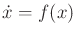is given along with an equilibrium point,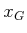. Can the various forms of stability be easily determined? One of the most powerful methods to prove stability is to construct a Lyapunov function. This will be introduced shortly, but first some alternatives are briefly mentioned.

If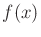is linear, which means that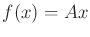for some constant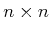matrix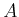and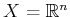, then stability questions with respect to the origin,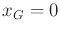, are answered by finding the eigenvalues of. The state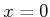is asymptotically stable if and only if all eigenvalues ofhave negative real parts. Consider the scalar case,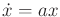, for which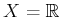and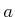is a constant. The solution to this differential equation is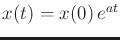, which converges to 0 only if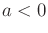. This can be easily extended to the case in whichandis andiagonal matrix for which each diagonal entry (or eigenvalue) is negative. For a general matrix, real or complex eigenvalues determine the stability (complex eigenvalues cause oscillations). Conditions also exist for Lyapunov stability. Every equilibrium state of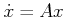is Lyapunov stable if the eigenvalues ofall have nonpositive real parts, and the eigenvalues with zero real parts are distinct roots of the characteristic polynomial of.

Ifis nonlinear, then stability can sometimes be inferred by linearizingaboutand performing linear stability analysis. In many cases, however, this procedure is inconclusive (see Chapter 6 of ). Proving the stability of a vector field is a challenging task for most nonlinear systems. One approach is based on LaSalle's invariance principle [39,156,585] and is particularly useful for showing convergence to any of multiple goal states (see Section 5.4 of ). The other major approach is to construct a Lyapunov function, which is used as an intermediate tool to indirectly establish stability. If this method fails, then it still may be possible to show stability using other means. Therefore, it is a sufficient condition for stability, but not a necessary one.

Subsections
Steven M LaValle 2020-08-14